## Exercises

1. Prove that the Cartesian product of a metric space is a metric space by taking a linear combination as in (5.4).
2. Prove or disprove: If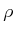is a metric, then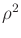is a metric.
3. Determine whether the following function is a metric on any topological space::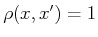is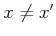; otherwise,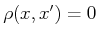.
4. State and prove whether or not (5.28) yields a metric space on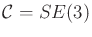, assuming that the two sets are rigid bodies.
5. The dispersion definition given in (5.19) is based on the worst case. Consider defining the average dispersion: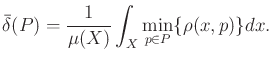(5.42)

Describe a Monte Carlo (randomized) method to approximately evaluate (5.42).
6. Determine the average dispersion (as a function of) for the van der Corput sequence (base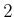) on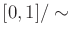.
7. Show that using the Lebesgue measure on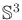(spreading mass around uniformly on) yields the Haar measure for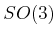.
8. Is the Haar measure useful in selecting an appropriate C-space metric? Explain.
9. Determine an expression for the (worst-case) dispersion of theth sample in the base-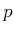(Figure 5.2 shows base-2) van der Corput sequence in, in which 0 andare identified.
10. Determine the dispersion of the following sequence on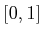. The first point is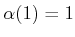. For each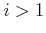, let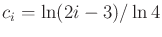and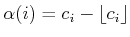. It turns out that this sequence achieves the best asymptotic dispersion possible, even in terms of the preceding constant. Also, the points are not uniformly distributed. Can you explain why this happens? [It may be helpful to plot the points in the sequence.]
11. Prove that (5.20) holds.
12. Prove that (5.23) holds.
13. Show that for any given set of points in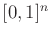, a range space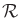can be designed so that the discrepancy is as close as desired to.
14. Suppose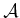is a rigid body in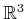with a fixed orientation specified by a quaternion,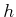. Suppose thatis perturbed a small amount to obtain another quaternion,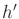(no translation occurs). Construct a good upper bound on distance traveled by points on, expressed in terms of the change in the quaternion.
15. Design combinations of robots and obstacles in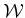that lead to C-space obstacles resembling bug traps.
16. How many-neighbors can there be at most in an-dimensional grid with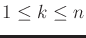?
17. In a high-dimensional grid, it becomes too costly to consider all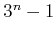-neighbors. It might not be enough to consider only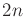1-neighbors. Determine a scheme for selecting neighbors that are spatially distributed in a good way, but without requiring too many. For example, what is a good way to select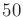neighbors for a grid in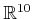?
18. Explain the difference between searching an implicit, high-resolution grid and growing search trees directly on the C-space without a grid.
19. Improve the bound in (5.31) by considering the fact that rotating points trace out a circle, instead of a straight line.
20. (Open problem) Prove there are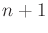main branches for an RRT starting from the center of an infinite''-dimensional ball in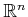. The directions of the branches align with the vertices of a regular simplex centered at the initial configuration.

Implementations

21. Implement 2D incremental collision checking for convex polygons to obtain near constant time'' performance.
22. Implement the sampling-based roadmap approach. Select an appropriate family of motion planning problems: 2D rigid bodies, 2D chains of bodies, 3D rigid bodies, etc.
1. Compare the roadmaps obtained using visibility-based sampling to those obtained for the ordinary sampling method.
2. Study the sensitivity of the method with respect to the particular NEIGHBORHOOD method.
3. Compare random and deterministic sampling methods.
4. Use the bridge test to attempt to produce better samples.
23. Implement the balanced, bidirectional RRT planning algorithm.
1. Study the effect of varying the amount of intermediate vertices created along edges.
2. Try connecting to the random sample using more powerful descent functions.
3. Explore the performance gains from using Kd-trees to select nearest neighbors.
24. Make an RRT-based planning algorithm that uses more than two trees. Carefully resolve issues such as the maximum number of allowable trees, when to start a tree, and when to attempt connections between trees.
25. Implement both the expansive-space planner and the RRT, and conduct comparative experiments on planning problems. For the full set of problems, keep the algorithm parameters fixed.
26. Implement a sampling-based algorithm that computes collision-free paths for a rigid robot that can translate or rotate on any of the flat 2D manifolds shown in Figure 4.5.

Steven M LaValle 2020-08-14